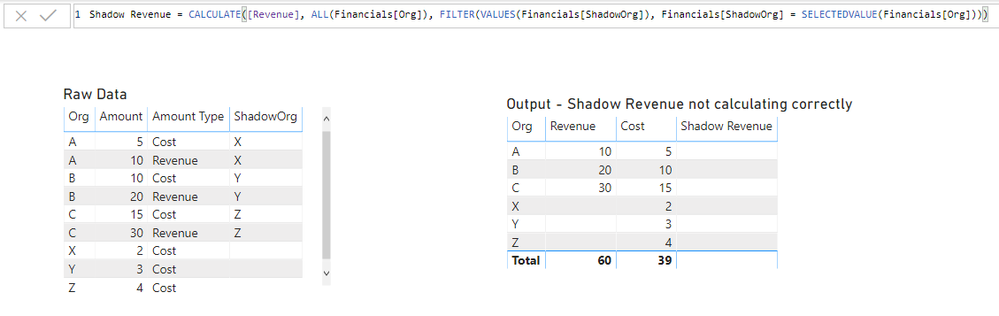cancel
Showing results for
Did you mean:Helper V

## Filter by another column in a measure

The sample data below is kind of a representation of my data in it's simplest form.

 Org Amount Amount Type ShadowOrg A 10 Revenue X A 5 Cost X B 20 Revenue Y B 10 Cost Y C 30 Revenue Z C 15 Cost Z X 2 Cost Y 3 Cost Z 4 Cost

I mainly have 3 metrics I'm trying to get from it. The first 2, Revenue and Cost are straightfoward.

Revenue = CALCULATE(SUM(Financials[Amount]), Financials[Amount Type] = "Revenue")
Cost = CALCULATE(SUM(Financials[Amount]), Financials[Amount Type] = "Cost")

For Shadow Revenue, I want to be able to show the revenue based on the field ShadowOrg but I want to show it in a table that has the Org as one of the columns.
Here's what I've set it up as but it doesn't work.I expect to see the following values in the Shadow Revenue Column
X = 10
Y = 20
Z = 30

What am I doing wrong?

4 REPLIES 4Community Champion

Hi @vickyd

try a measure

``````Shadow Revenue = CALCULATE([Revenue],
)``````

do not hesitate to give a kudo to useful posts and mark solutions as solution

do not hesitate to give a kudo to useful posts and mark solutions as solutionHelper V

Thanks @az38  for the quick response.

Although your solution worked, I think I over-simplified my sample data. In reality, Financials is my fact table and I have several dimension tables associated to it in a star schema structure. In my sample, imagine a OrgMaster and ShadowOrgMaster table exists along with maybe few other dimension tables.

I do not want to remove all the filters from the Financial FACT table, only want to remove any filters from the OrgMaster table when calculating the Shadow Revenue.

So something like this wouldn't work giving a syntax error.

)Community Champion

if  you are trying to add a measure into ShadowOrgMaster it should look like

``````Shadow Revenue = CALCULATE([Revenue],
)``````

do not hesitate to give a kudo to useful posts and mark solutions as solution

do not hesitate to give a kudo to useful posts and mark solutions as solutionHelper V

@az38 wrote:

if  you are trying to add a measure into ShadowOrgMaster it should look like

``````Shadow Revenue = CALCULATE([Revenue],
)``````

do not hesitate to give a kudo to useful posts and mark solutions as solution

OrgMaster will not have ShadowOrg column and ShadowOrgMaster will not have the Org column.

Not seeing an attach file option here and have restrictions for file sharing at work else would have shared the PBIX file. Will try to share once I'm home.